# Building a Compute Block Tutorial¶In this tutorial, we will build a compute block in Arundo Edge to calculate the total power output of two wind turbines.

The tutorial is divided into the following sections:

• Learning Objectives - things you will know how to do after completing the tutorial.
• Setup - set up necessary before you get started.
• Instructions - instructions on how to complete the tutorial.
• Finishing Up - challenges and further reading for after the tutorial.

## Learning Objectives¶

After completing this tutorial, you will know how to:

• Create simulators that stream sample data
• Build compute blocks that calculate custom values
• View the output of compute blocks

## Setup¶

Before you start the tutorial, you must do the following:

## Instructions¶

Now let's get started...

### 1. Set up a Simulator¶

The first step is to set up a simulator to stream sample turbine data.Sample Turbine Data

Save the file on your local device.

Tip

The simulator will stream data from this file.

#### b) Add a Sequence Interface¶

Add a Sequence interface in Arundo Edge:

Tip

The Sequence interface will stream the sample data into Arundo Edge on repeat.

1. Double-click the Arundo Edge shortcut on the desktop.
3. Select Interfaces | + Add new.
4. Select Sequence.
5. In Name, enter "Turbine Simulator".

#### c) Upload the Sample Data¶

Upload the sample data file to Sequence interface by clicking Upload CSV (highlighted below).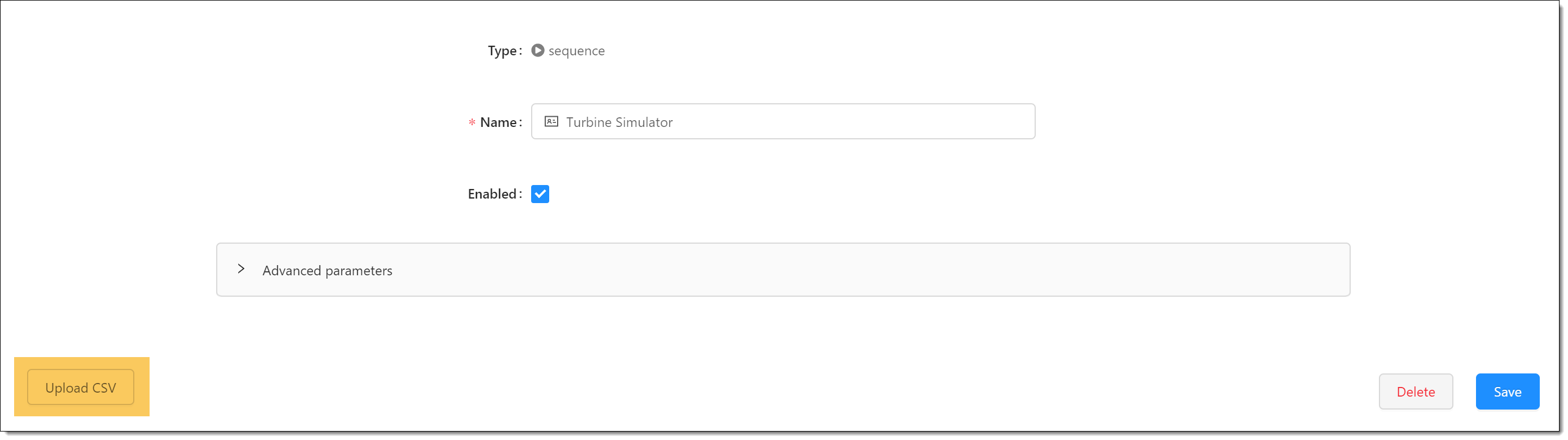Then click the upload box and select the sample data file. The sample data now displays on the screen as follows:### 2. Build a Compute Block¶

The next step is to build a compute block on the Compute Chain screen. To do this, select Compute Chain on the sidebar and click Add Compute Block.

#### a) Enter Basic Details¶

Enter basic details for the compute block, including the name, update criteria, and refresh interval.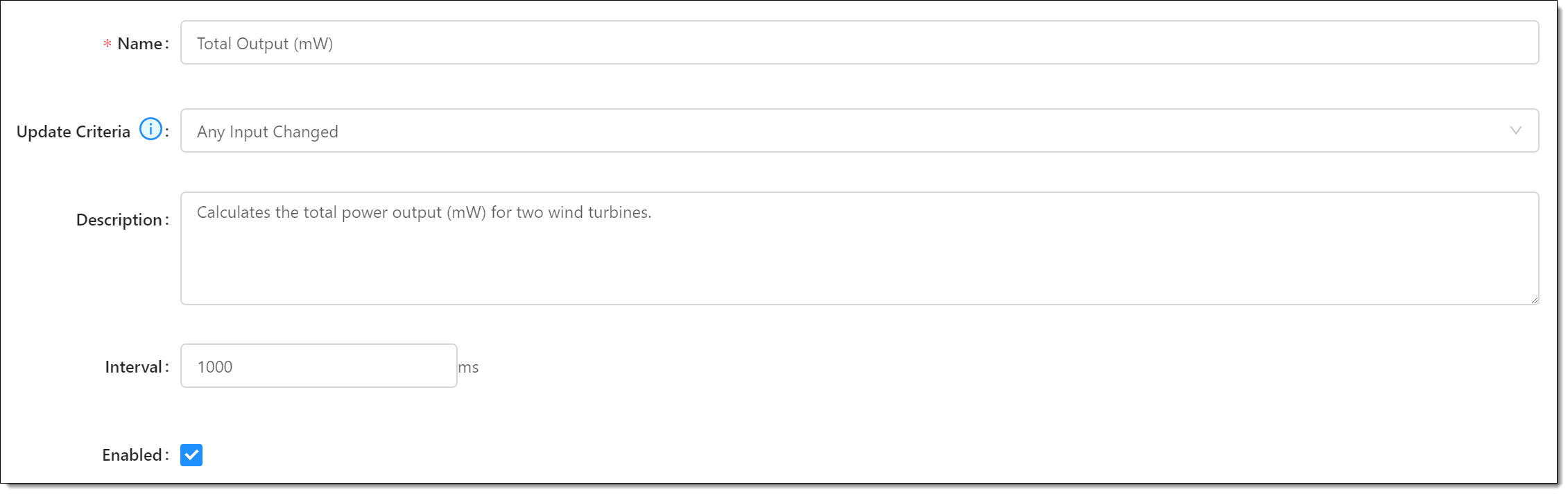Move down to the Inputs section and add the tags from the simulator we set up in Step 1: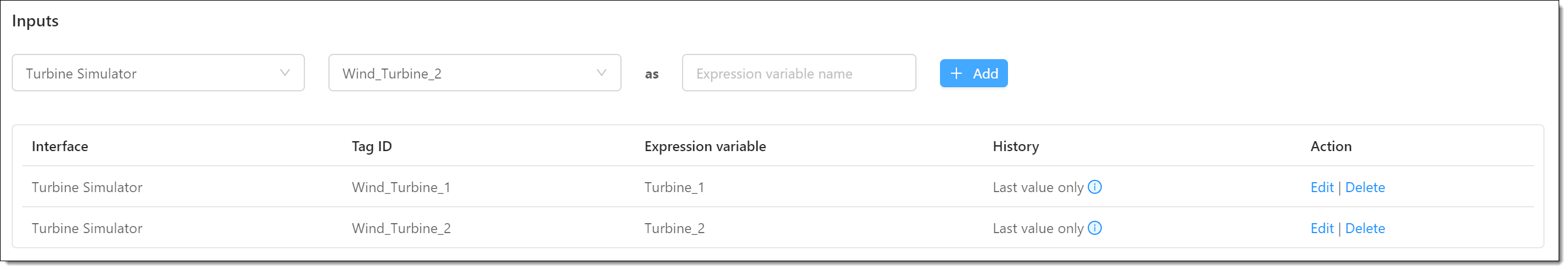#### c) Add an Output Tag¶

Next, move down to the Outputs section and add an output tag to store the value calculated by the compute block: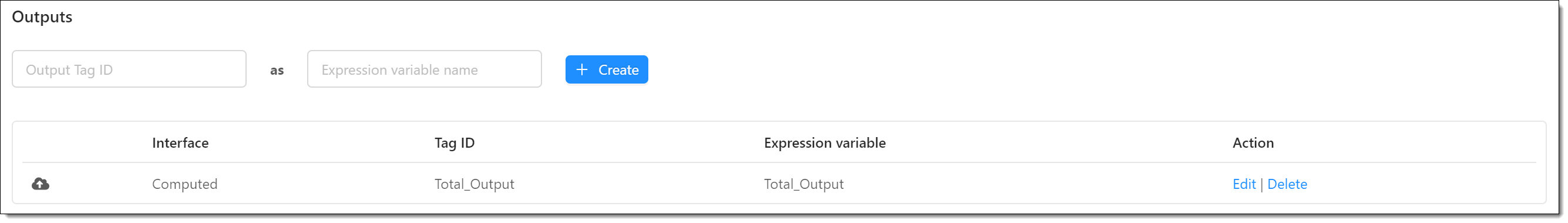#### d) Define an Expression¶

Define the following expression to calculate the value of the Total_Output tag: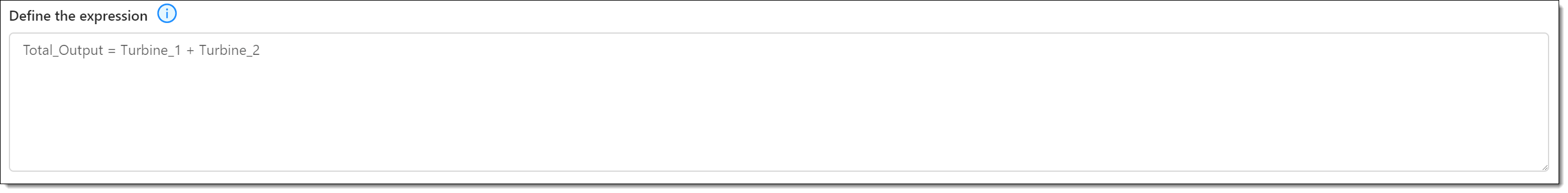Tips

• Enter the output tag first in the expression.

Finally, click Save to add the compute block.

### 3. View the Output¶

The final step is to view the calculated output for the compute block. To do this, complete the following:

1. Select Viewer on the sidebar.
2. Open the Graph tab.
3. Select "Local" in Filter.
4. Select Auto refresh.

The output displays on the Total_Output panel under Compute: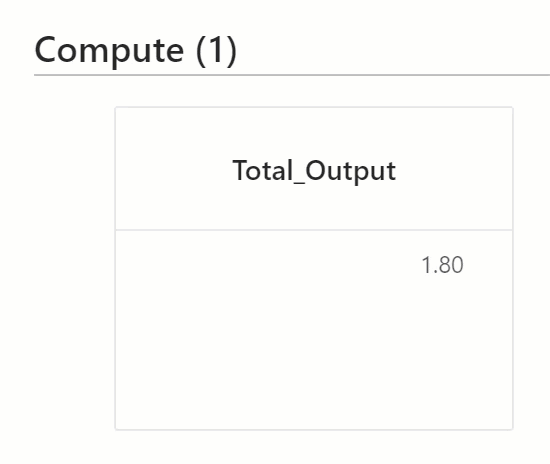## Finishing Up¶

Congratulations! You've now built a compute block that performs a simple calculation. We hope you now understand how to use compute blocks to enhance your data analytics.

Want to expand your knowledge further? Try the following:

Challenge

Build your own compute blocks to calculate custom tag values.Sample Turbine Data